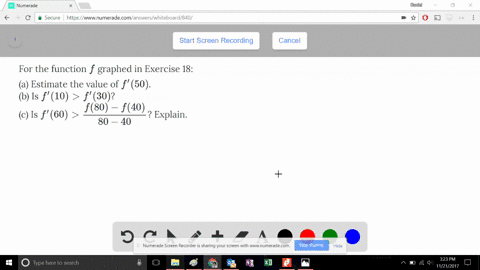Enroll in one of our FREE online STEM summer camps. Space is limited so join now!View Summer Courses### For the function $f$ graphed in Exercise 18: …

03:07

Need more help? Fill out this quick form to get professional live tutoring.

Get live tutoring
Problem 18

The graph of a function $f$ is shown.

(a) Find the average rate of change of $f$ on the interval $[20, 60]$.
(b) Identify an interval on which the average rate of change of $f$ is 0.
(c) Which interval gives a larger average rate of change, $[40, 60]$ or $[40, 70]$?
(d) Compute $\dfrac{f(40) - f(10)}{40 - 10}$; what does this value represent geometrically?

a) 10
b) Rate of change is 0 on the interval $[10,50]$
c) Rate of change is higher on the interval $[40,60]$
d) see solution

## Discussion

You must be signed in to discuss.

## Video Transcript

this problem. Number eighteen of the sewer calculus eighth edition Section two point seven The graph of function after shown party on the average rate of change of F on the interval, twenty to sixteen. So this is the graph of the function F shown in the book. Find the average rate of change of F on the interval twenty two sixty. So, in order to do average rate of change, we used his function here, showing at the top where we have the function evaluated at the latter part of the Interval Dynasty function. Value it at the beginning part of interval, divided by the difference between ah x two and six one or sixteen minutes. Twenty. So what is sixty evaluated at its approximately seven hundred and twenty is approximately three hundred. So if we were to calculate this for party, our average rate of change is about seven hundred, minus three hundred divided by sixty minus twenty to Benjamin is three hundred is four hundred sixty minutes twenty. It's forty seven hundred exchanges four hundred sixty minutes. Twenty is forty grand. Dad wrote about forty is ten Terrible great change is ten for party Party identified in a role in which the average rate of change of F zero well, our average it'll change function is here. It can only be zero if the function of the function of the end of the interval is the same as the function on to being the integral. That's when these two will cancel. So the function is equivalent. Um, definitely at the ten. So at him for X, we have four hundred and we also have four hundred four fifty. So, uh, one interval ten to fifty. What? Haven't average rate of change of zero. It's a party. Our answer is ten two fifteen party. Which animal gives a larger average rate of change? Forty two. Sixty or forty to seventy. Forty two, sixty years. Approximately going from two hundred two, seven hundred over sixty. Minus forty seven minus two hundred is five hundred five hundred. Divided by twenty is approximately twenty five now for the second into rules from forty to seventy. It goes all the way up to nine hundred for seventy That's two hundred The writer by seventy minus forty. So this is my underwent A seven hundred which is nine hundred minus two hundred to seven hundred Get out of my seventy minus forty, which is thirty seven hundred already bright thirties, approximately twenty three three. So here it is clearly clear to see the internally gives. Largest large average rate of change is the interval from forty to sixty because it will have an overall larger slopes party. Compute This average rate of change in the Inderal tend to forty after forty on the graph is we can see is two hundred f of ten. As we can see, it's four hundred. So two hundred minus four hundred over forty minutes. Ten. Here we have two hundred miles for injured. His negative two hundred. And down here we have thirty forty minutes. Ten. Yes, sir. This is to native twenty over there. Three. What is this Value Represent geometrically. All this represents the slope of the secret line between half of ten and have a forty. And this is the average rate of change of the function F for the interval from ten to forty